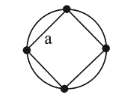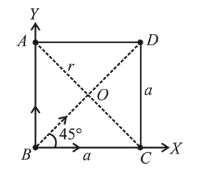# Four identical particles of mass M

Question:

Four identical particles of mass $\mathrm{M}$ are located at the corners of a square of side ' $a$ '. What should be their speed if each of them revolves under the influence of others' gravitational field in a circular orbit circumscribing the square ?1. (1) $1.35 \sqrt{\frac{\mathrm{GM}}{a}}$

2. (2) $1.16 \sqrt{\frac{\mathrm{GM}}{a}}$

3. (3) $1.21 \sqrt{\frac{\mathrm{GM}}{a}}$

4. (4) $1.41 \sqrt{\frac{\mathrm{GM}}{a}}$

Correct Option: , 2

Solution:

(2) $A C=a \sqrt{2} \quad \because r=\frac{A C}{2}=\frac{a \sqrt{2}}{2}=\frac{a}{\sqrt{2}}$

Resultant force on the body

$B=\frac{G M^{2}}{a^{2}} \hat{i}+\frac{G M^{2}}{a^{2}} \hat{j}+\frac{G M^{2}}{(a \sqrt{2})^{2}}$

$\left(\cos 45^{\circ} \hat{i}+\sin 45^{\circ} \hat{j}\right)$

$\Rightarrow|F|=\frac{G M^{2}}{a^{2}}(\sqrt{2})+\frac{G M^{2}}{2 a^{2}}$$\frac{M v^{2}}{r}=$ Resultant force towards centre

$\therefore \frac{M v^{2}}{\left(\frac{a}{\sqrt{2}}\right)}=\frac{G M^{2}}{a^{2}}\left(\sqrt{2}+\frac{1}{2}\right)$

$\Rightarrow v^{2}=\frac{G M}{a}\left(1+\frac{1}{2 \sqrt{2}}\right)$

$\Rightarrow v=\sqrt{\frac{G M}{a}\left(1+\frac{1}{2 \sqrt{2}}\right)}=1.16 \sqrt{\frac{G M}{a}}$